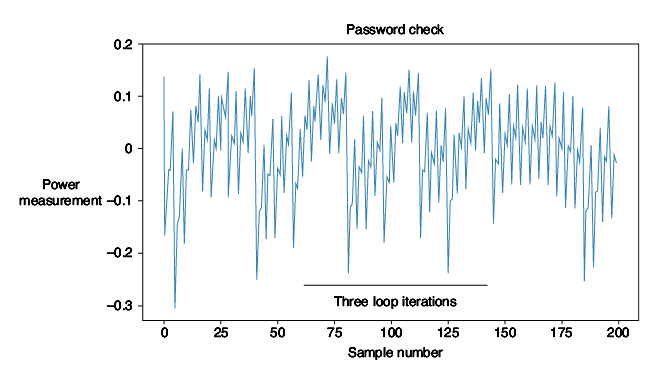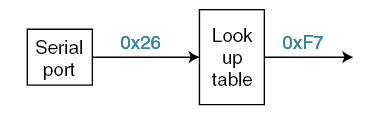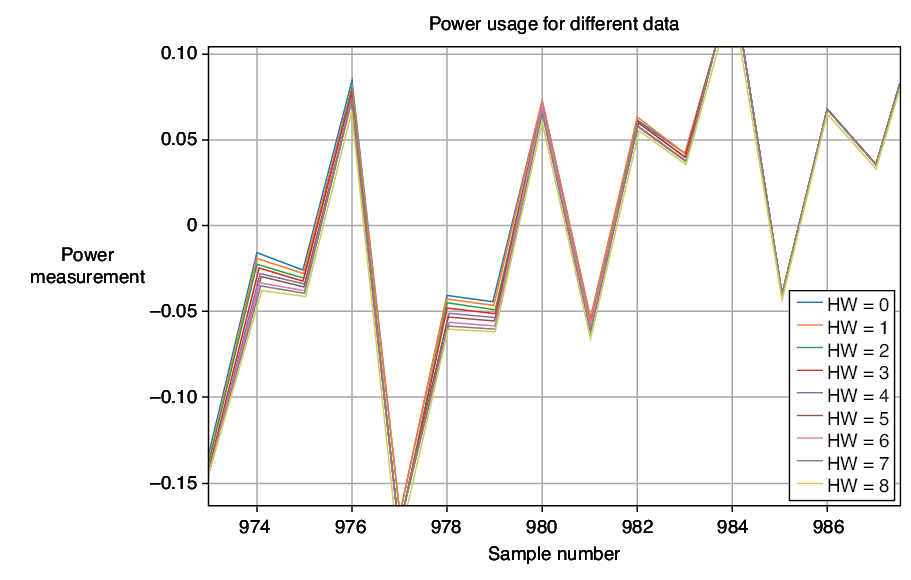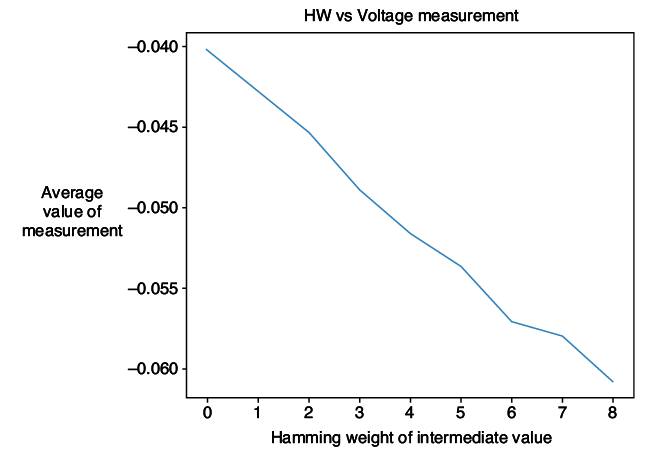# Side-Channel Power AnalysisWritten by

## Easy Path to Proof

This month I thought I’d bring you an introduction to side-channel power analysis (again). I’ve covered this in past articles, but it’s been a few years and I know new readers are going to be picking up this issue of Circuit Cellar. But don’t worry—I’m doing more than just giving you a rehash of old material. My open-source ChipWhisperer project has recently had the Version 5.0 release, which uses a new interactive Python interface (using something called Jupyter notebooks). As part of this release, several new tutorials are available, and some of them cover aspects I haven’t previously shown you.

In particular, I’m going to show you how some of the fundamental assumptions around side-channel power analysis can be easily proven. It’s not something for which you have to take my word. It’s something you can test yourself, and experiment with the differences that show up for various firmware code you might be running.

SIMPLE POWER ANALYSIS
My intro is going to push through all sorts of examples. The first thing we’ll talk about is simple power analysis (SPA). This form of power analysis commonly refers to the fact that you can see the flow of data through a system. This can be used to break code that has an execution path that depends on the secret data being processed. What sort of code might that be? We’ll take a look at a simple password check as shown in Listing 1. That might look straight-forward—but what if you could see the loop execution time? Power analysis lets us do exactly that, meaning that we could discover which character of our password was incorrect.

`char passwd; char correct_passwd[] = “h0px3”; my_read(passwd, 32);`

``` uint8_t passbad = 0; trigger_high();— ADVERTISMENT— if (document.avp_ready) { avp_zone({ base: 'linuxgizmos.advertserve.com', type: 'banner', zid: 39, pid: 3 }); } —Advertise Here— ```

`for(uint8_t i = 0; i < sizeof(correct_passwd); i++){ if (correct_passwd[i] != passwd[i]){ passbad = 1; break; } }`

LISTING 1
An example of a simple password check, where if one could figure out the loop count, one could recover the password byte-by-byte.

The code from Listing 1 also contains a `trigger_high() `and `trigger_low()` call. Those actually provide an added piece of instrumentation being used only for our demonstration. Using a resistor in the power pin, we could see how the power is varying, as in Figure 1. I’m doing that with my ChipWhisperer platform, but you could use an oscilloscope or other similar piece of gear. You can see in Figure 2 the loop has an obvious pattern, and we see four iterations through the loop.FIGURE 1Power consumption can be measured with a resistor in the VCC line of the device. Here I’ve also removed decoupling capacitors to improve the strength of the signal. We AC-couple the measurement to remove the high-DC bias, since we are looking at small variations only.FIGURE 2A power trace of the loop execution helps you understand how many iterations through the loop your code ran, which could break the password check in Listing 1.

How does that help us crack a password? We could monitor the power consumption of the device and send every possible first character of the password. When we see a change in the power trace, we know that suddenly another code path was taken. Most likely this “other code path” is in fact the loop going into the second iteration. We don’t need to be clever or look for a specific signature. We just look for “different.”

It’s hard to hide this difference. If we add a random delay afterward, we can still see the time at which the power traces changed. We can notice that at this point in time whether it seems to go into a busy-wait loop or continues processing data. If you don’t believe me, there is an exact example of this in the open-source ChipWhisperer Jupyter.

So, if you think you’re clever, you’ll implement the code as in Listing 2. This takes the same amount of time, no matter what code is executed. Let’s see how to break that.

`char passwd; char correct_passwd[] = “h0px3”; my_read(passwd, 32);`

``` uint8_t passbad = 0;— ADVERTISMENT— if (document.avp_ready) { avp_zone({ base: 'linuxgizmos.advertserve.com', type: 'banner', zid: 39, pid: 3 }); } —Advertise Here— trigger_high(); ```

`for(uint8_t i = 0; i < sizeof(correct_passwd); i++){ passbad |= correct_passwd[i] ^ passwd[i]; }`

LISTING 2
An example of a time-independent password check that could be broken by looking at power consumption of the device.

DATA AFFECTS POWER
What if I told you that the very data being processed affect the power consumption? The theory behind this is fairly simple. Internal to the device, a data bus consists of wires over a ground plane. Changing the voltage on this is equivalent to charging and discharging a capacitor. As a nice feature, most internal data-buses go to an intermediate state between valid data transmissions. These intermediate states mean that every time we send a value across the data-bus, we have to charge a certain number of data lines to the ‘1’ state. If we looked at the power consumed on the VCC rail, we would expect to see spikes related to the data being sent across a bus. If all the bits of the data-bus were going high, we would expect to see larger spikes than when only one or two lines went high. You can see our expected results in Figure 3.FIGURE 3Different numbers of bits being set to ‘1’ on the power trace result in different power consumption on each clock cycle.

But how could we test that? We could send some data to a chip, and try to find a location where, for example, we see a strong difference in the power being used that depends on the data. Since we expect our signal to be very weak, we might need to average a number of such traces over time.

Let’s try that experiment! To do this, I’m actually going to target a specific area of an encryption algorithm. Why not just plot the location of the received data directly? While we could do that, there is a good chance we’d trick ourselves into thinking the signal is stronger than it really is. In particular, if I use a serial interface to send 0xFF on repeat, I would also expect a strong signal because this serial interface is being driven to one level! So instead, I’m going to use something like the setup in Figure 4, where the input data are passed through a random look-up table. This look-up table is reversible, which is to say that every one of the 256 inputs maps to only one output.FIGURE 4A very simple piece of code that simplifies our validation of the power consumption is related to number of bits set to one on a data bus.

Including this look-up table means that to get a certain number of ones at the output, we are sending an unrelated number of ones in the input. For example, to get seven bits set to one, we could look at the output 0xF7 or 0x7F. In our particular look-up table that would mean that 0x26 and 0x6b, respectively, get sent—those with 3 bits and 5 bits. Therefore, the messages going over the serial port wouldn’t have the same number of bits set. As a result, we wouldn’t expect to see as much influence on our signal from just the serial data line.

How do we know where the actual output is? Our evaluation path will be to send hundreds or even thousands of random bytes to firmware that is executing the code in Figure 4. Because we know the input to the look-up table—we sent it after all—we know the output of the look-up table. This means we could put every possible trace into a “group” based on the number of ones in the output of the look-up table. This number of ones will be the “Hamming weight” of the data.

VALIDATION TIME
Based on my claims from Figure 3, I would expect that at some point in time, there would be a measurable difference in the power consumption. Let’s try to validate that now. If we plot the average of each group, there is a point where the averages noticeably diverge, as in Figure 5. The final confirmation is to plot the value at this point in time versus the Hamming weight of the data, which has an almost linear fit (Figure 6). Note that we are actually measuring a one-sided voltage drop across a shunt resistor, which we AC-couple to remove the DC bias. Importantly, if we were drawing no current, we actually would have a zero voltage. As we increase our instantaneous current draw, we will see a higher negative value due to the AC-coupling. Therefore, in this graph we see a lower voltage measurement for more bits set to one. It’s a minor detail, but in case you were wondering why things are “flipped,” there is a reason behind it.FIGURE 5The average power consumption for different output Hamming weights (bits set to one) of the look-up table in Figure 4.FIGURE 6We can see a strong linear relationship between the number of bits set to one on the bus (Hamming weight) and the power consumption at a specific instant in time.

What is really interesting is that all “other” points have very little difference. This makes sense if you consider that any part not within our data of interest has been effectively randomly sorted into different groups. If you take the average of each group, it makes sense that the average power consumption at these other points is the same. So, we not only see the relationship between data being processed and power consumption, but we also see the point in time where our look-up table was used.

You might wonder how this works for an attack. It turns out the start of the AES algorithm looks very much like Figure 4, except that, instead of just taking the input data, the input data is XOR’d with a byte of the secret key (Figure 7). We know from Figure 5 that we can detect the averages diverging if we group each power trace into the correct Hamming weight, and from Figure 6 that there is a strong linear relationship.FIGURE 7The beginning of the real AES algorithm involves a secret key being XOR’d with some input data, before being passed through a look-up table (Substitution Box).

The “attack” is that we can recover bytes of the encryption key, one byte at a time. This means we can break a 16-byte AES-128 or a 32-byte AES-256 key in reasonable time—even just a few minutes. This is done by sending random (known) input data. We don’t need to observe the output of the algorithm in this example.

We could then guess every possibility for 1 byte of the secret key marked in Figure 7. For each of the 256 possibilities for this byte, we could perform the same procedure and group power traces into “possible” Hamming weight groups. If our byte of the secret key matches the real secret key byte, we should be able to create plots similar to those in Figure 5 and Figure 6. If the guessed key does not match, we expect the average of each power trace to overlap as it did for all non-interesting positions.

A GOOD MATCH?
We can quantify how good the “match” is simply by looking for the linear relationship that we found from our experiment that created Figure 6. This is done by measuring the correlation between Hamming weight and power at each point in time. The correlation can be thought of as a measure of how close to a straight line the data points create. Plotting the correlation over time for each guess, you would see that at the same point in time that we saw the output of the lookup table, we also see a strong correlation. This strong correlation suggests that there is a solution to the problem of “what value of the secret key would allow us to properly group the power traces?”

The solution to that problem becomes a single byte of the encryption key. We can repeat this for each successful byte, and suddenly you’ve broken an AES-128 encryption key. This simple idea underpins one way that side-channel power analysis works to derive encryption keys by monitoring only the input data and the power consumption. The fun part is trying to prove the basics yourself. If you need more hints, check out the ChipWhisperer 5.0 release, which includes several Jupyter-based tutorials that will help you recreate the figures used in my article—except you’ll be recreating them with real measurements, not just simulations or assumptions.

Additional materials from the author are available at:
www.circuitcellar.com/article-materials

RESOURCES
NewAE Technology | www.newae.com

PUBLISHED IN CIRCUIT CELLAR MAGAZINE • MARCH 2019 #344 – Get a PDF of the issue

 Keep up-to-date with our FREE Weekly Newsletter! Don't miss out on upcoming issues of Circuit Cellar. Subscribe to Circuit Cellar Magazine Note: We’ve made the May 2020 issue of Circuit Cellar available as a free sample issue. In it, you’ll find a rich variety of the kinds of articles and information that exemplify a typical issue of the current magazine. Would you like to write for Circuit Cellar? We are always accepting articles/posts from the technical community. Get in touch with us and let's discuss your ideas.